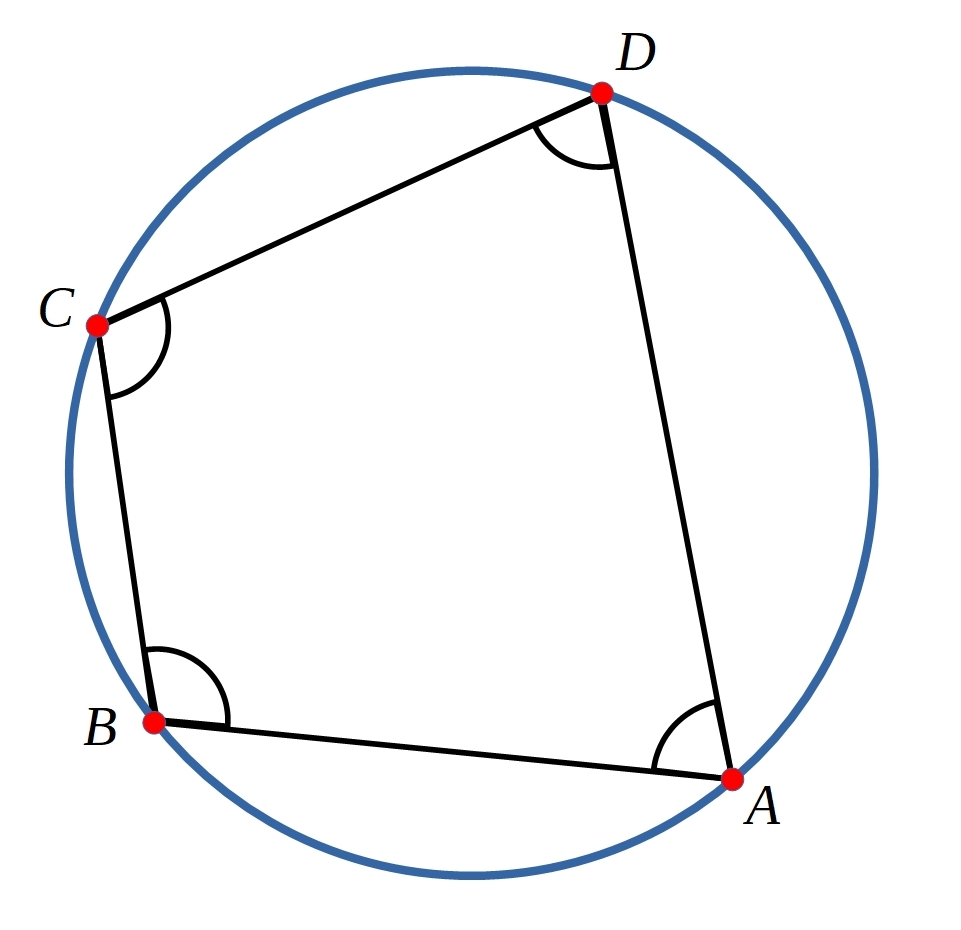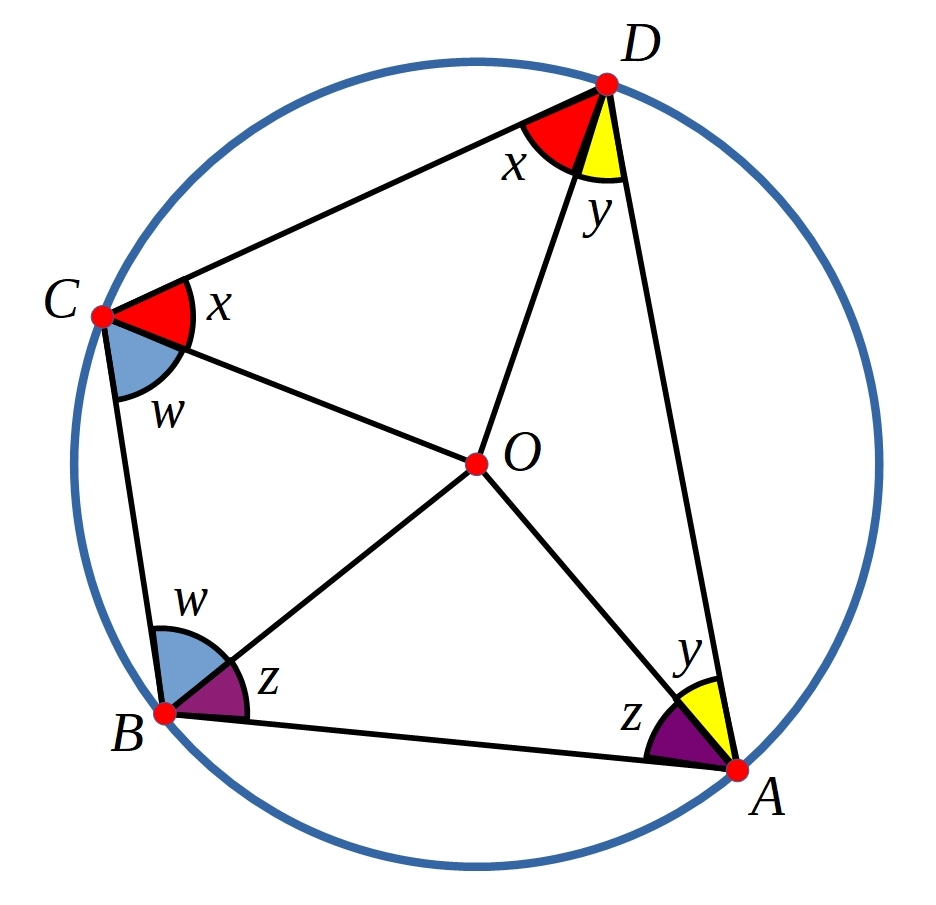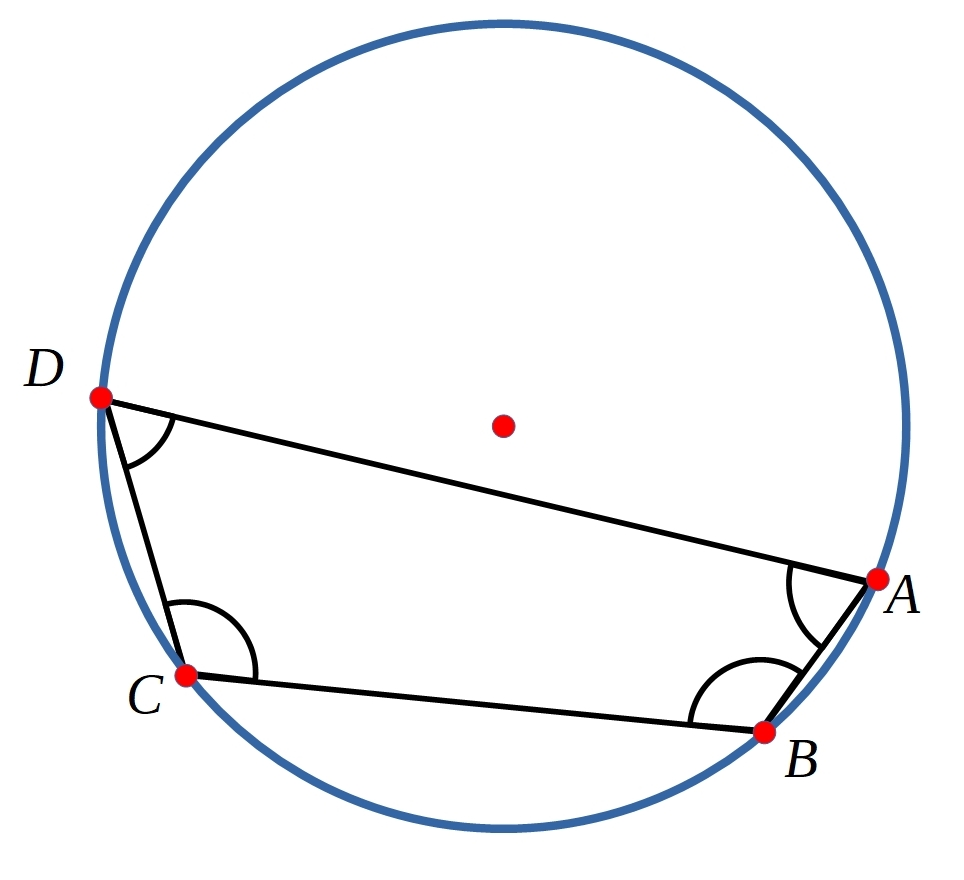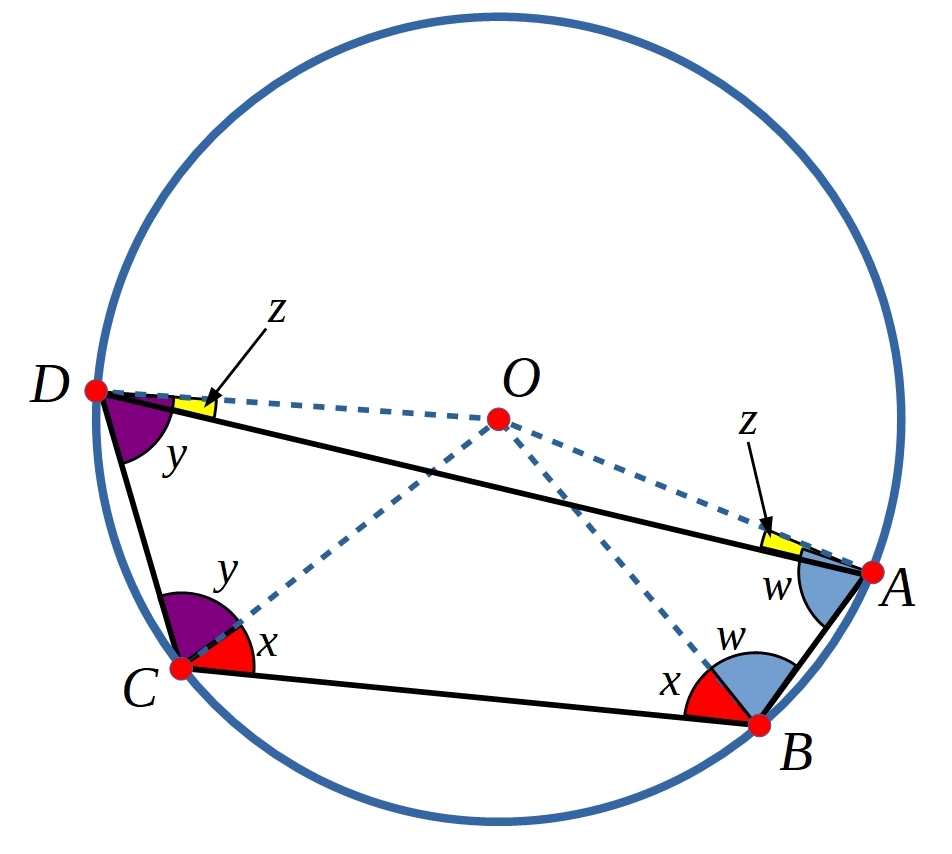##### Age 11 to 16Challenge LevelThis problem follows on from Cyclic Quadrilaterals

Sketch a circle and choose four points at random to form a quadrilateral.

Can you prove that the opposite angles of your quadrilateral add to $180^\circ$?

Click below to see a diagram that might help you to prove it.Quadrilaterals whose vertices lie on the edge of a circle are called Cyclic Quadrilaterals.

ExtensionCan you prove that when the centre of the circle is outside the cyclic quadrilateral, the opposite angles also add to $180^\circ$?

You may wish to draw some examples on
9, 10, 12, 15 and 18 dot circles.

Click below to see a diagram that might help you to prove that the opposite angles of cyclic quadrilaterals add to $180^\circ$, when the centre of the circle is outside the cyclic quadrilateral.You may be interested in the other problems in our Sharpen your Skills Feature.

We are very grateful to the Heilbronn Institute for Mathematical Research for their generous support for the development of this resource.